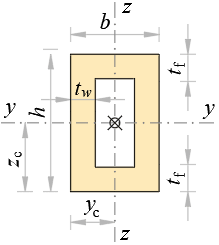### Geometrical Properties of Rectangular TubeDimensions

h = m, tw = m

b = m, tf = m

b1 = b – 2·tw m

h1 = h – 2·tf m

Area

A = 2·(h·tw + b1·tf) m2

A = h·bh1·b1 m2

Centroid

yc = b2 m

zc = h2 m

Perimeter

P = 2·(h + b) m

Second moments of area

Iy = b·h3b1·h1312 m4

Iz = b3·hb13·h112 m4

Polar moment of area

Ix = Iy + Iz m4